# Backpropagation(反向传播)方法介绍2019年5月6日07:57:20

4435字阅读14分47秒

## 数学基础

### Chain Rule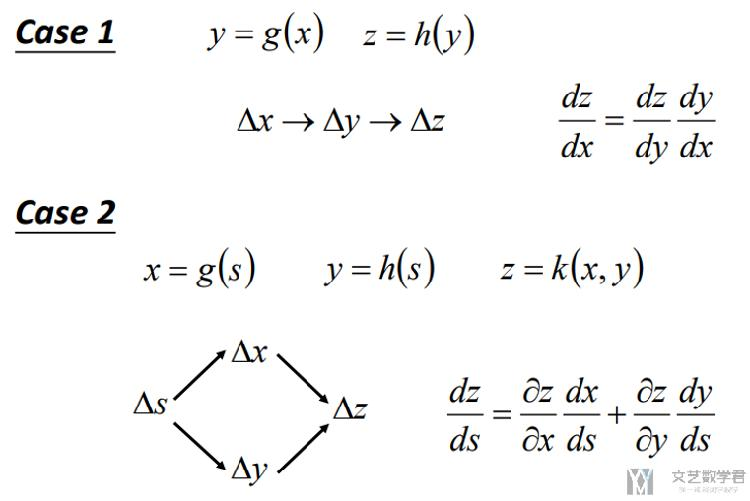### Sigmoid函数导数## 简单例子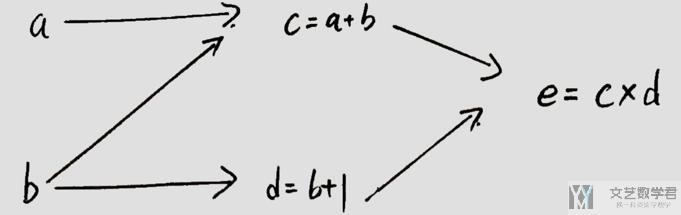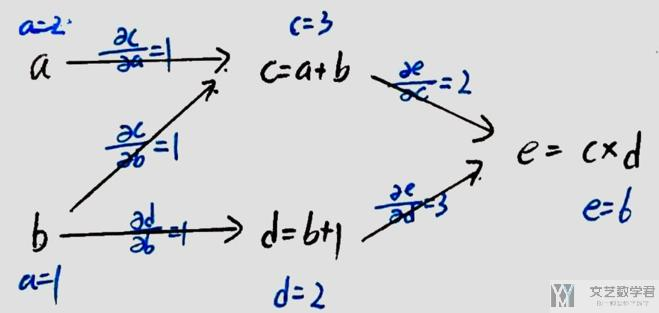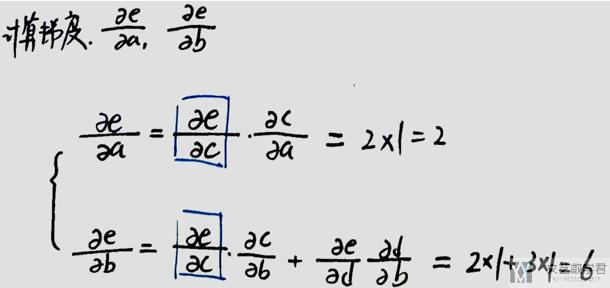### 使用链式法则的一些问题

• 如求e关于b的偏导,需要找出e到b的所有路径, 正向求导比较麻烦;
• 一些值会重复使用, 具体看下面的一个例子.

## 全链接网络推导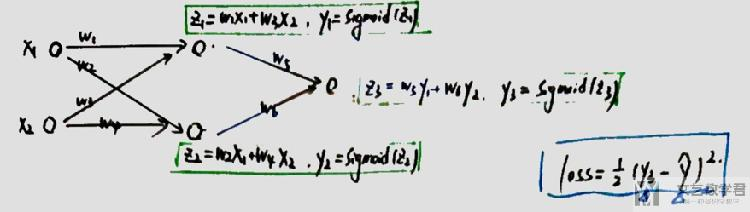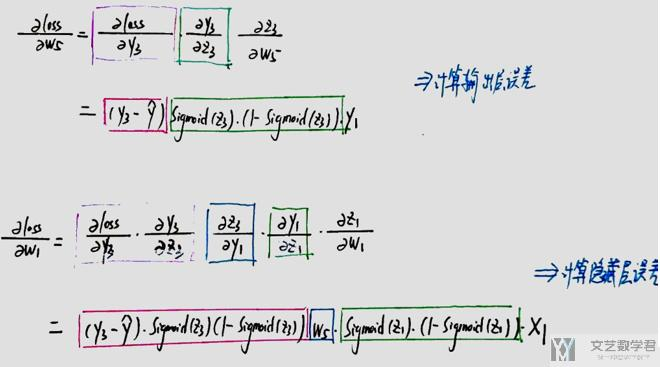## 计算图与反向传播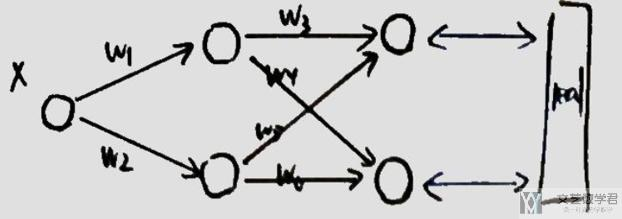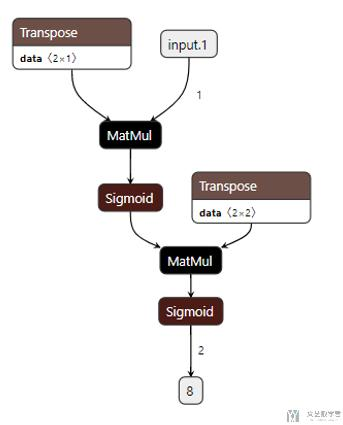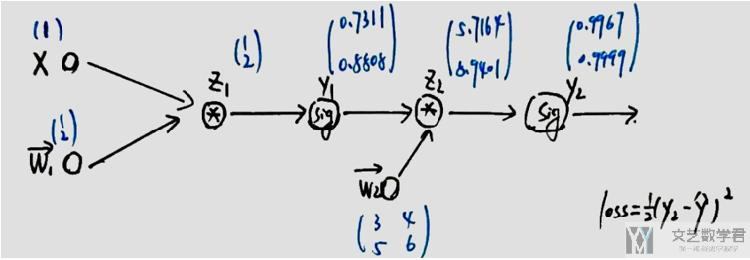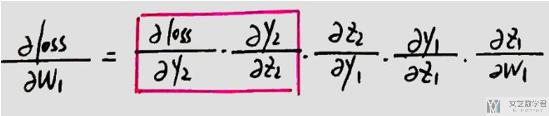• 相当于从loss开始往前传递梯度.
• 会重复利用之前计算的梯度, 如上图中红色框出的部分.
• 上图中红色框出的部分, 在计算loss对w2的偏导时, 计算完毕后会存储在起来(可以理解为存储在z2节点处), 下次在计算loss对w1的偏导时, 可以重复利用该值.

## Pytorch实验

### 网络的定义

1. class NeuralNet(nn.Module):
2.     def __init__(self):
3.         super(NeuralNet, self).__init__()
4.         self.linear1 = nn.Linear(in_features=1, out_features=2, bias=False)
5.         self.linear2 = nn.Linear(in_features=2, out_features=2, bias=False)
6.         self.sig = nn.Sigmoid()
7.     def forward(self, x):
8.         out = self.linear1(x)
9.         print(out)
10.         out = self.sig(out)
11.         # y1 = out.detach().numpy().reshape(2,1)
12.         print(out)
13.         out = self.linear2(out)
14.         print(out)
15.         out = self.sig(out)
16.         # y2 = out.detach().numpy().reshape(2,1)
17.         print(out)
18.         # 可以自己尝试自己写一下梯度的计算
21.         return out

### 初始化系数

1. # 网络的初始化
2. model = NeuralNet()
3. # 原始的weight
4. for num, (name, params) in enumerate(model.named_parameters()):
5.     print(num,params.data.shape,'\n',params.data)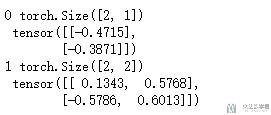1. # 做初始化系数
2. model.linear1.weight.data = torch.FloatTensor([,])
3. # model.linear1.bias.data = torch.FloatTensor([1,2])
4. model.linear2.weight.data = torch.FloatTensor([[3,4],[5,6]])
5. # model.linear2.bias.data = torch.FloatTensor([1,2])
6. # 现在的系数
7. for num, (name, params) in enumerate(model.named_parameters()):
8.     print(num,params)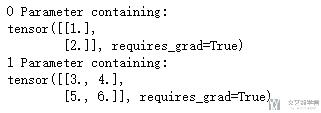### 正向传播

1. # 正向传播
2. input_data = Variable(torch.FloatTensor())
3. out = model(input_data)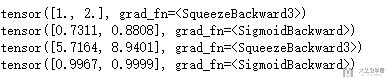1. # 计算误差
2. label = Variable(torch.FloatTensor([1,0]))
3. loss_fn = nn.MSELoss(reduction='mean')
4. loss = loss_fn(out, label)
5. loss

1. # 打印梯度和系数
4. # 现在的系数
5. for num, (name, params) in enumerate(model.named_parameters()):
6.     print(num,params)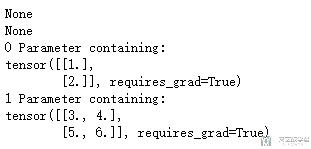### 反向传播

1. # 反向传播
2. loss.backward()
3. # 打印梯度和系数
6. # 现在的系数
7. for num, (name, params) in enumerate(model.named_parameters()):
8.     print(num,params)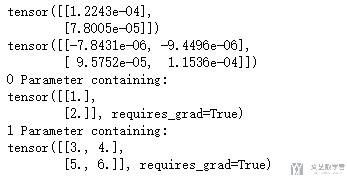### 更新weight

1. # 更新参数参数
2. optimiser = optim.SGD(params=model.parameters(), lr=1) # 定义优化器
3. optimiser.step()

### 梯度清零, 进行下一次计算loss

1. # 梯度清零
3. # 打印梯度和系数
6. # 现在的系数
7. for num, (name, params) in enumerate(model.named_parameters()):
8.     print(num,params)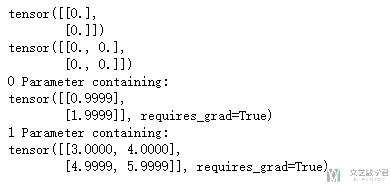### 导出模型并绘图

1. # 打印网络结构
2. import torch.onnx
3. # 输入测试数据
4. input_data = Variable(torch.FloatTensor())
5. torch.onnx.export(model, input_data, "model2.onnx", export_params=True, verbose=True, training=True)

## 梯度消失

• 当y的绝对值取值较大时, Sigmoid的导数会趋于0
• 可以看下面的导数的图像
• 也可以理解为其导数为(1-z)*z, z容易趋于0或是1, 都会使导数趋于0
• 同时, (1-z)*z的最大值为1/4, 在z=1/2时取到, 也就是说每经过一个Sigmoid激活函数, 梯度会变为原来的1/4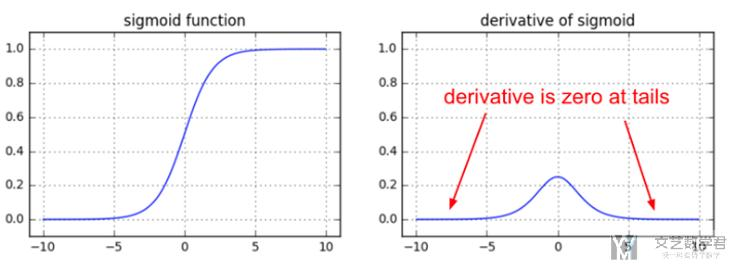## 关于Pytorch中backward的一点说明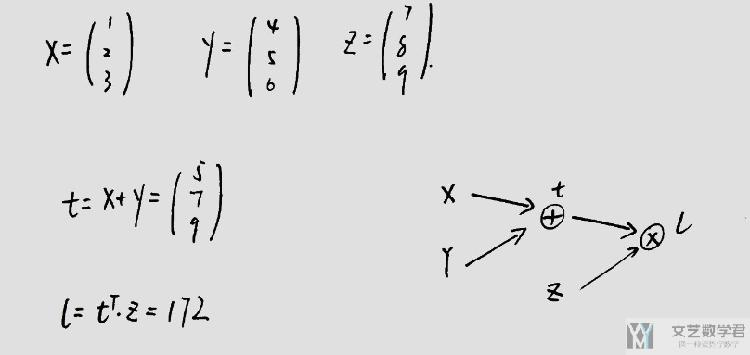4. t = x + y
5. l = torch.mm(t.reshape(1,3),z)

### 直接进行l.backward()

1. l.backward()
2. # 打印梯度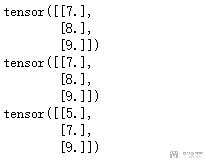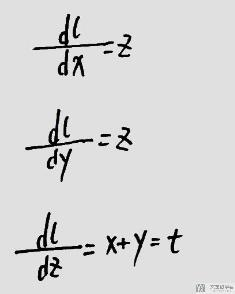### 进行t.backward(z)进行反向传播

1. # 这个等价于l.backward()
2. t.backward(z)
3. # 打印梯度## 参考文章• Yes you should understand backprop[Andrej Karpathy]
• Getting Started with PyTorch Part 1: Understanding how Automatic Differentiation works

• 微信公众号
• 关注微信公众号
•• QQ群
• 我们的QQ群号
•• 本文由 发表于 2019年5月6日07:57:20
• 转载请务必保留本文链接：https://mathpretty.com/10409.html